• 周志华《机器学习》中的BP神经网络有其变量的推导，很详细，我在以下给出几页推导过程。我在我的资源里上传了PDF版的，谁要的话可以下载。 以下是我的代码，仅供参考，大部分是矩阵直接运算 clear clc ...
学习了周志华《机器学习》中的BP神经网络，编代码的时候想找别人的作参考，发现好多人都直接调用工具箱。所得非所求，只能是自己写了代码，仅供新手参考，其中大部分是矩阵直接运算，对鸢尾花2/3数据训练，1/3数据训练。准确率在96%左右。
说明：代码的直接矩阵运算也是我坐那里耗费一些时间自己去想的，所以新手要看懂可能需要一些时间。一些人可能没读过这本书也会感觉云里雾里，鉴于篇幅问题，我在我的资源了上传了直接运行的代码、数据集和《机器学习》相应的理论说明，那里面有详细的梯度项理论推导，地址如下：https://download.csdn.net/download/weixin_44405310/11530170 有书的人自然心领神会。
以下是我的一些学习心得：
**什么是梯度下降法：**在这里我推一篇博文：https://blog.csdn.net/pengchengliu/article/details/80932232 大家可以参考，讲这个的人很多。
数据归一化问题： 有人不理解为什么要数据归一化，我举一个浅显的例子：一个属性集的数据数量级是10$^4$,它的阈值是0.1；另一个属性集的数据数量级是10，就算它的阈值是1，把它们分别乘起来；我们发现数量级是10的那个属性集分明就是鸡肋嘛！影响不了大局。
而matlab自带归一化函数mapminmax好像只能对行归一化，我不想转置，就自己写了一段程序。
分类： 鸢尾花是要分类的，那么输出神经元该怎么办。我们可以这样，看它有几个类别，那就定义几个输出神经元。对本例而言：[0 1 0]就可以说是第二类，刚开始的类别标签也要这么处理。当然计算的输出神经元不可能那么准确，但是非常接近1。总之，就是取最大的位置作为它的类别。其实我还有一个想法，就是把最后的激活函数乘3，只设置一个输出神经元，如果输出是0~1就是第一类， 输出在1~2就判定第二类。
隐层神经元的数目我是随便设的，这个我也不知道设置多少。
clear
clc
f=fopen('C:\Users\HP\Desktop\iris.data');
Iris=textscan(f,'%f%f%f%f%s','delimiter',',');
fclose(f);
[~,n]=size(Iris);
for i=1:n-1
data(:,i)=Iris{1,i};
end
[m,n]=size(data);
for i=1:m
if strcmp(Iris{1,5}{i,1},'Iris-setosa')
data(i,5:7)=[1 0 0];
elseif  strcmp(Iris{1,5}{i,1}, 'Iris-versicolor')
data(i,5:7)=[0 1 0];
else
data(i,5:7)=[0 0 1];
end
end
%数据归一化处理[0,1]区间内,
x_max=max(data);
x_min=min(data);
data=(data-ones(m,1)*x_min)./(ones(m,1)*(x_max-x_min));
% 这里可用函数data=mapminmax(data,0,1)替换
%但data需要转置因为mapminmax只能对行进行归一化
%%
%划分训练样本和测试样本 大约2/3用作训练，1/3用作测试
num=round(m/3/3);
for i=1:3
temp=data(1+50*(i-1):50*i,:);
sel=randperm(50,num);
test(1+num*(i-1):num*i,:)=temp(sel,1:4);
test_label(1+num*(i-1):num*i,:)=temp(sel,5:7);
temp(sel,:)=[];
train(1+(50-num)*(i-1):(50-num)*i,:)=temp(:,1:4);
train_label(1+(50-num)*(i-1):(50-num)*i,:)=temp(:,5:7);
end
%%
[m,n]=size(train);
alpha=4;%输入神经元数目
beta=8;%隐层神经元数目
lamda=3;%输出神经元数目
rng('shuffle')
W1=rand(alpha,beta);%输入层和隐层之间的权值矩阵
W2=rand(beta,lamda);%隐层和输出层间的权值矩阵
B1=rand(1,beta);%隐层阈值矩阵
B2=rand(1,lamda);%输出层阈值矩阵
B22=B2;
W11=W1;
Eta=1;%学习率
iter_max=10000;%最大迭代次数
iter=1;
%BP神经网络，每次仅针对一个训练样例更新连接权和阈值
while iter<=iter_max
for i=1:m
hidden_in=train(i,:)*W1;%隐层输入
hidden_out=sigmod(hidden_in-B1);%隐层输出
output_in=hidden_out*W2;%输出层输入
output_out=sigmod(output_in-B2);%输出层输出
%计算误差
E(i)=sum((output_out-train_label(i,:)).^2);%求平方和可用sumsqr函数
%%
%更新参数,BP神经网络中最核心的部分
g=output_out.*(1-output_out).*(train_label(i,:)-output_out);%计算输出层神经元的梯度项
e=hidden_out.*(1-hidden_out).*(g*W2');%计算隐层神经元的梯度项
Deta_W2=Eta*hidden_out'*g;
Deta_B2=-Eta*g;
Deta_W1=Eta*train(i,:)'*e;
Deta_B1=-Eta*e;
W1=W1+Deta_W1;
W2=W2+Deta_W2;
B1=B1+Deta_B1;
B2=B2+Deta_B2;
end
%计算训练集的累积误差
E=mean(E);
if E<1e-4  %目标误差
iter
break
end
if mod(iter,1000)==0
iter
end
iter=iter+1;%更新迭代次数
end
%%
%测试
[result,accuracy]=BP_test(test,test_label,W1,W2,B1,B2);
disp('结果为：')
result';
disp(strcat('准确率为',num2str(accuracy*100),'%'))
function [result,accuracy]=BP_test(test,test_label,W1,W2,B1,B2)
%返回分类的结果
%accuracy准确率
Hidden_in=test*W1;%隐层输入
Hidden_out=sigmod(Hidden_in-B1);%隐层输出
Output_in=Hidden_out*W2;%输入层输入
Output_out=sigmod(Output_in-B2);
[~,result]=max(Output_out,[],2);
[~,index2]=max(test_label,[],2);
right=sum(result==index2);%统计分类正确的个数
total=size(test,1);%总个数
accuracy=right/total;
end
%sigmod函数在matlab里是logsig函数
function [Y]=sigmod(X)
Y=1./(1+exp(-1).^X);
end

这是结果：
ans =
1 至 19 列
 1     1     1     1     1     1     1     1     1     1     1     1     1     1     1     1     1     2     2

20 至 38 列
 2     2     2     3     2     2     3     2     2     2     2     2     2     2     2     3     3     3     3

39 至 51 列
 3     3     3     3     3     3     3     3     3     3     3     3     3

准确率为96.0784%


展开全文• 本代码主要利用MATLAB工具进行MATLAB——有导师学习神经网络分类——鸢尾花种类识别
• 鸢尾花识别经典算法有很多，处于分类的一块的知识，对于分类的技术也有很多很多，通过BP神经网络也可以实现，在这里介绍一种更好的神经网络来实现鸢尾花的识别问题。
• MATLAB平台下实现构造前馈神经网络，实现BP算法，对鸢尾花数据集进行分类BP算法
• 有导师学习神经网络分类 ---鸢尾花种类识别的理论基础，案例背景，MATLAB程序实现
• matlab关于ann的分类方法讲解了一个例子，Fishr集上鸢尾花（Iris）的分类，学习了这个方法可以套用在个人项目上使用，万变不离其宗， 1、Fishr集上鸢尾花Iris数据集的分类 ①iris数据集简介 iris数据集的中文名是...
matlab关于ann的分类方法讲解了一个例子，Fishr集上鸢尾花（Iris）的分类，学习了这个方法可以套用在个人项目上使用，万变不离其宗，

1、Fishr集上鸢尾花Iris数据集的分类
①iris数据集简介
iris数据集的中文名是安德森鸢尾花卉数据集，英文全称是Anderson’s Iris data set。iris包含150个样本，对应数据集的每行数据。每行数据包含每个样本的四个特征和样本的类别信息，所以iris数据集是一个150行5列的二维表。
通俗地说，iris数据集是用来给花做分类的数据集，每个样本包含了花萼长度、花萼宽度、花瓣长度、花瓣宽度四个特征（前4列），我们需要建立一个分类器，分类器可以通过样本的四个特征来判断样本属于山鸢尾、变色鸢尾还是维吉尼亚鸢尾（这三个名词都是花的品种）。
iris的每个样本都包含了品种信息，即目标属性（第5列，也叫target或label）。

样本局部截图：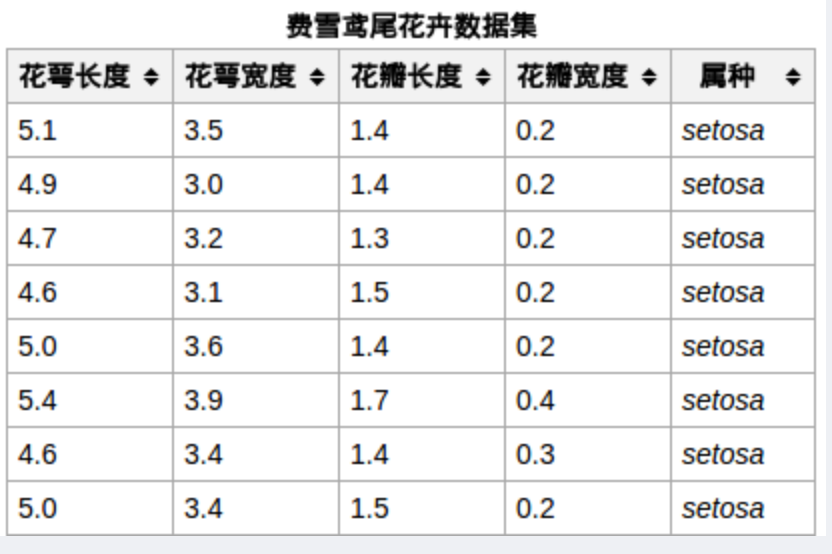load iris.dat

将数据集载入到工作区，部分数据集如图所示。数据集的前四列分别为与鸢尾花种类相关的4个特征值，对应上图中的花萼长度、花萼宽度、花瓣长度及花瓣宽度；第五列为鸢尾花所属种类，分为1-Setosa、2-Versicolour、3-Virginica三类。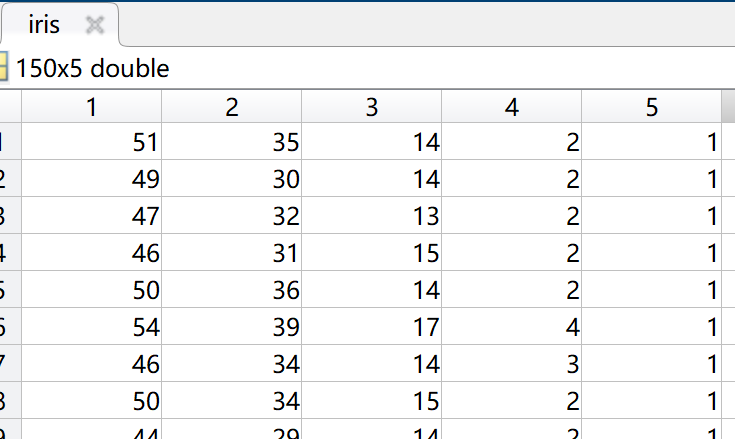②数据预处理
这里的神经网络属于监督学习的模式，因此需要从上述数据集中分离出训练集和测试集，我们分别记为trainData和testData。我们从iris数据集中选取2/3数据作为训练集trainData，选取1/3数据作为测试集testData，并分别将其保存至trainData.txt和testData.txt文件，用于程序的数据导入源，方法见另外一个博客（https://blog.csdn.net/qingfengxd1/article/details/105931988）

③分类源程序

%读取训练数据
clear
clc
%------本代码采用ANN对鸢尾花进行分类，程序运行前，请准备好#鸢尾花样本#的训练集和测试集（可在MATLAB中载入iris.dat查看数据）-----
%f1 f2 f3 f4是四个特征值
[f1,f2,f3,f4,class] = textread('trainData.txt' , '%f%f%f%f%f',150);
%特征值归一化
[input,minI,maxI] = premnmx( [f1 , f2 , f3 , f4 ]')  ;
%构造输出矩阵
s = length( class) ;
output = zeros( s , 3  ) ;
for i = 1 : s
output( i , class( i )  ) = 1 ;
end
%创建神经网络
net = newff( minmax(input) , [10 3] , { 'logsig' 'purelin' } , 'traingdx' ) ;
%{
minmax(input)：获取4个输入信号（存储在f1 f2 f3 f4中）的最大值和最小值；
[10,3]：表示使用2层网络，第一层网络节点数为10，第二层网络节点数为3；
{ 'logsig' 'purelin' }：
表示每一层相应神经元的激活函数；
即：第一层神经元的激活函数为logsig（线性函数），第二层为purelin（对数S形转移函数）
'traingdx'：表示学习规则采用的学习方法为traingdx（梯度下降自适应学习率训练函数）
%}
%设置训练參数
net.trainparam.show = 50 ;% 显示中间结果的周期
net.trainparam.epochs = 500 ;%最大迭代次数（学习次数）
net.trainparam.goal = 0.01 ;%神经网络训练的目标误差
net.trainParam.lr = 0.01 ;%学习速率（Learning rate）
%开始训练
%其中input为训练集的输入信号，对应output为训练集的输出结果
net = train( net, input , output' ) ;
%================================训练完成====================================%
%=============================接下来进行测试=================================%

%读取测试数据
[t1 t2 t3 t4 c] = textread('testData.txt' , '%f%f%f%f%f',150);

%测试数据归一化
testInput = tramnmx ( [t1,t2,t3,t4]' , minI, maxI ) ;
%[testInput,minI,maxI] = premnmx( [t1 , t2 , t3 , t4 ]')  ;
%仿真
%其中net为训练后得到的网络，返回的Y为
Y = sim( net , testInput )

%统计识别正确率
[s1 , s2] = size( Y ) ;
hitNum = 0 ;
for i = 1 : s2
[m , Index] = max( Y( : ,  i ) ) ;
if( Index  == c(i)   )
hitNum = hitNum + 1 ;
end
end
sprintf('识别率是 %3.3f%%',100 * hitNum / s2 )

④代码的相关说明
A. 语句net = newff( minmax(input) , [10 3] , { 'logsig' 'purelin' } , 'traingdx' ) ;用于创建神经网络，其参数含义和用法如下：

（1）minmax(input)：获取4个输入信号（存储在f1 f2 f3 f4中）的最大值和最小值；
（2） [10,3]：表示使用2层网络，第一层网络节点数为10，第二层网络节点数为3。其中最后一层的网络包含的节点数一定要与网络的理论输出个数保持一致，例如本例中鸢尾花的种类数为3，因此最后一层的网络节点数为3；
（3）{ 'logsig' 'purelin' }：表示每一层相应神经元的激活函数，即：第一层神经元的激活函数为logsig（线性函数），第二层为purelin（对数S形转移函数），其他激活函数和用法请参见神经网络与深度学习之激活函数；
（4） 'traingdx'：表示学习规则采用的学习方法为traingdx（梯度下降自适应学习率训练函数）。常见的训练函数（学习方法）有：

traingd ：梯度下降BP训练函数(Gradient descent backpropagation)
traingdx ：梯度下降自适应学习率训练函数

（5）创建的神经网络用MATLAB神经网络工具箱显示如图，图中更形象的展示了构造的神经网络模型。

B. 关于正确率的统计算法的说明

第一次看到这里的正确率统计算法时，我自己是不大明白的，之后又从网上搜了一些资料并查阅了MATLAB的帮助文档，才明白代码的含义。

语句net = train( net, input , output' ) ;是对网络进行训练，该语句明确了网络的输出为output，通过对output矩阵的构造方式分析，我们可知网络的输出可以看成3个，我们不妨即为C1、C2、C3，分别代表鸢尾花的三个种类，例如：

（1）当output的某一行为1 0 0，则说明该花属于C1类

（2）当output的某一行为0 1 0，则说明该花属于C2类

（3）当output的某一行为0 1 0，则说明该花属于C3类

语句Y = sim( net , testInput ) 是对训练后的网络net进行仿真测试，测试用的数据为testInput；这里，Y返回的是网络训练后对测试输入的预测值，例如：

（1）当Y的某一行为1.0220  -0.0020  -0.0091，代表输出结果C1=1.0220， 输出结果C2=-0.0020，C3=-0.0091

（2）当Y的某一行为-0.0108  0.9884  -0.0216，代表输出结果C1=-0.0108，输出结果C2=0.9884，C3=-0.0216

输出结果中只包含一个1和两个0是理想情况下的结果，在进行仿真时，分类输出往往达不到这样的结果，但我们可以根据哪个结果对应的值与1的接近程度来进行判断，例如仿真结果（1）说明该花极有可能属于C1类，仿真结果（2）说明该花极有可能属于C2类。

*从以上描述中我们可以明白神经网络算法也可以应用于具体数值的预测，且应用广泛。展开全文• 本程序主要给出神经网络算法的两个简单应用，分别是Fishr集上鸢尾花（Iris）的分类以及常见的分类问题-蠓虫分类，同时通过对两个分类程序（Code by MATLAB）进一步分析，加强对神经网络算法的理解。
• 我用nnstart训练了一个BP神经网络，用其自带的MATLAB Function将这个神经网络保存， 然后在指令里调用，用来做150组鸢尾花数据集里面其中一组数据的预测。 我训练的时候是用的[0 1 0 ]这种格式定义的分类也就是...人工智能
• 实验内容： 现采集到 150 组不同类型鸢尾花...从图中 大致可以看出，花瓣长度、花瓣宽度与鸢尾花类型间有较好的线性关系，而萼片长度、萼 片 宽度与鸢尾花类型间呈现出非线性的关系。 现要求： 1. 利用 GRNN 和 P
实验内容：

现采集到 150 组不同类型鸢尾花（Setosa、Versicolour 和 Virginica）的四种属性： 萼片长度、萼片宽度、花瓣长度和花瓣宽度，样本编号与四种属性的关系如图 9.1 所示（其 中，样本编号 1-50 为 Setosa，51-100 为 Versicolour，101-150 为 Virginica）。从图中 大致可以看出，花瓣长度、花瓣宽度与鸢尾花类型间有较好的线性关系，而萼片长度、萼 片 宽度与鸢尾花类型间呈现出非线性的关系。 现要求：

1. 利用 GRNN 和 PNN 分别建立鸢尾花种类识别模型，并对模型的性能进行评价。

2. 利用 GRNN 和 PNN 分别建立各个属性及属性组合与鸢尾花种类间的识别模型，并对 所建 模型的性能及运算时间进行对比分析，从而探求各个属性及属性组合与鸢尾花种类的相 关程度。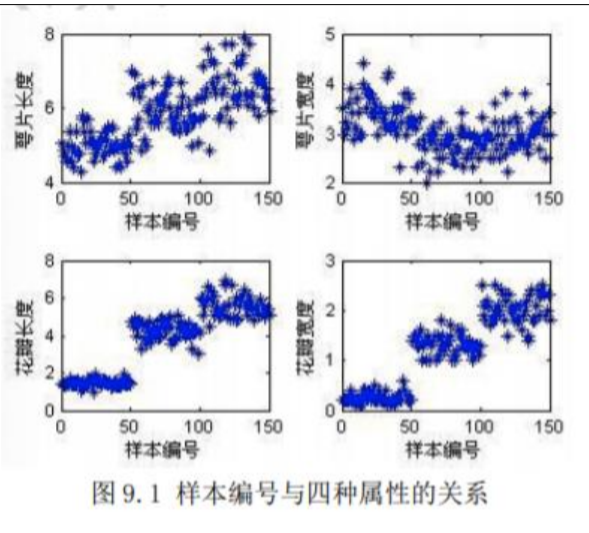直接上实现代码：

% 导入数据
load iris_data.mat
% 随机产生训练集和测试集
P_train = [];
T_train = [];
P_test = [];
T_test = [];
for i = 1:3
temp_input = features((i-1)*50+1:i*50,:);
temp_output = classes((i-1)*50+1:i*50,:);
n = randperm(50);
% 训练集——120 个样本
P_train = [P_train temp_input(n(1:40),:)'];
T_train = [T_train temp_output(n(1:40),:)'];
% 测试集——30 个样本
P_test = [P_test temp_input(n(41:50),:)'];
T_test = [T_test temp_output(n(41:50),:)'];
end
%% 模型建立
result_grnn = [];
result_pnn = [];
time_grnn = [];
time_pnn = [];
for i = 1:4
for j = i:4
p_train = P_train(i:j,:);
p_test = P_test(i:j,:);
%% GRNN 创建及仿真测试
t = cputime;
%创建网络
net_grnn = newgrnn(p_train,T_train);
%仿真测试
t_sim_grnn = sim(net_grnn,p_test);
T_sim_grnn = round(t_sim_grnn);
t = cputime - t;
time_grnn = [time_grnn t];
result_grnn = [result_grnn T_sim_grnn'];
%% PNN 创建及仿真测试
t = cputime;
Tc_train = ind2vec(T_train);
% 创建网络
net_pnn = newpnn(p_train,Tc_train);
% 仿真测试
Tc_test = ind2vec(T_test);
t_sim_pnn = sim(net_pnn,p_test);
T_sim_pnn = vec2ind(t_sim_pnn);
t = cputime - t;
time_pnn = [time_pnn t];
result_pnn = [result_pnn T_sim_pnn'];
end
end
%% 性能评价
%正确率 accuracy
accuracy_grnn = [];
accuracy_pnn = [];
time = [];
for i = 1:10
accuracy_1 = length(find(result_grnn(:,i) == T_test'))/length(T_test);
accuracy_2 = length(find(result_pnn(:,i) == T_test'))/length(T_test);
accuracy_grnn = [accuracy_grnn accuracy_1];
accuracy_pnn = [accuracy_pnn accuracy_2];
end
% 结果对比
result = [T_test' result_grnn result_pnn]
accuracy = [accuracy_grnn;accuracy_pnn]
time = [time_grnn;time_pnn]
%% 绘图
figure(1)
plot(1:30,T_test,'bo',1:30,result_grnn(:,4),'r-*',1:30,result_pnn(:,4),'k:^')
grid on
xlabel('测试集样本编号')
ylabel('测试集样本类别')
string = {'测试集预测结果对比(GRNN vs PNN)';['正确率:'
num2str(accuracy_grnn(4)*100) '%(GRNN) vs ' num2str(accuracy_pnn(4)*100)
'%(PNN)']};
title(string)
legend('真实值','GRNN 预测值','PNN 预测值')
figure(2)
plot(1:10,accuracy(1,:),'r-*',1:10,accuracy(2,:),'b:o')
grid on
xlabel('模型编号')
ylabel('测试集正确率')
title('10 个模型的测试集正确率对比(GRNN vs PNN)')
legend('GRNN','PNN')
figure(3)
plot(1:10,time(1,:),'r-*',1:10,time(2,:),'b:o')
grid on
xlabel('模型编号')
ylabel('运行时间(s)')
title('10 个模型的对比(GRNN vs PNN)')
legend('GRNN','PNN')

实验结果：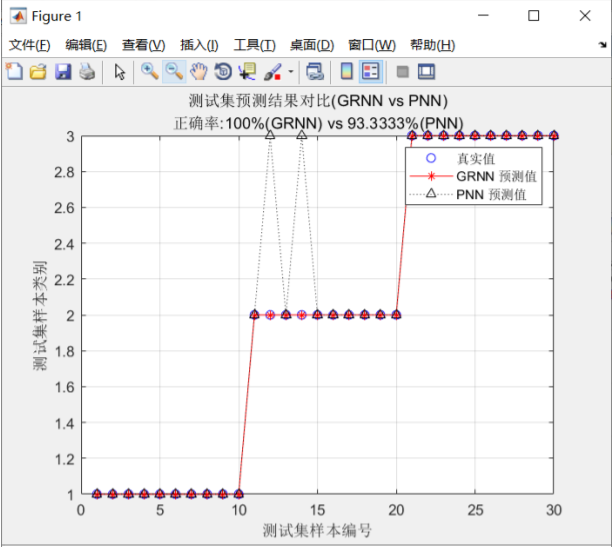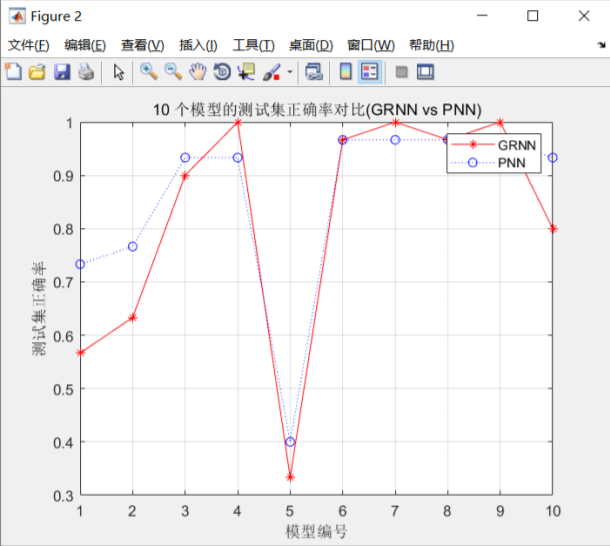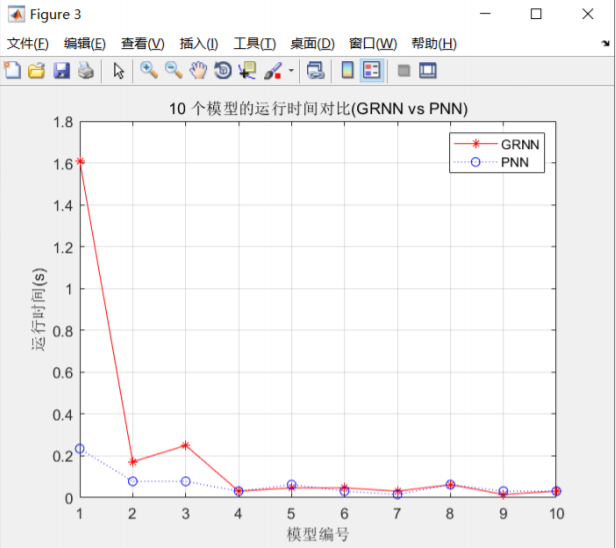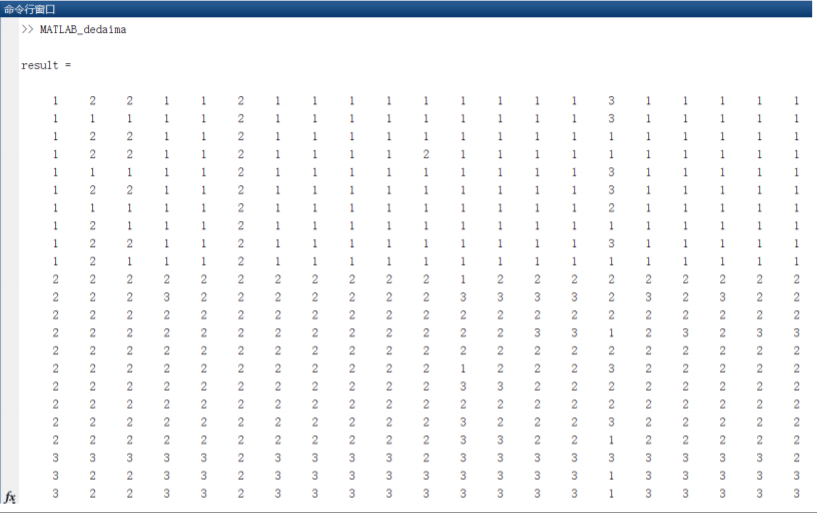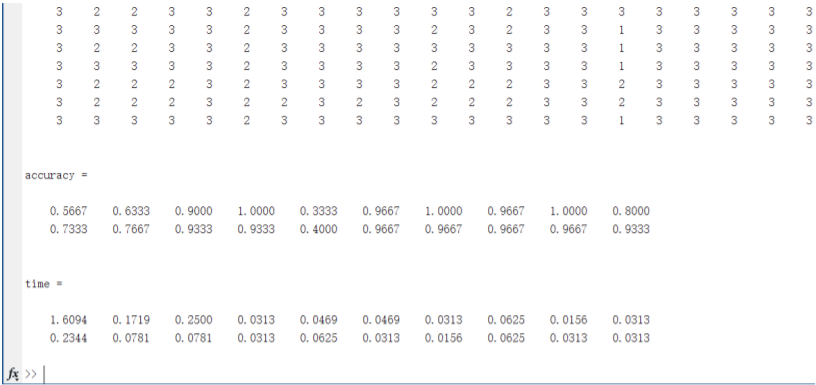实验总结：

通过这次实验，学习了 GRNN 和 PNN 神经网络的区别。之前学习了 BP 神经网络，我发现最 大的区别是 BP 神经网络是反向传播的，而且代码实现起来较为复杂，而 GRNN 和 PNN 神经网络 不是。对比 GRNN 神经网络和 PNN 神经网络我发现在时间上来说 GRNN 神经网络在第一次的运行 时间较长，但后面就会渐渐变短，GRNN 网络相比较于 GNN 神经网络来说准确率却会更高一些。 能够更好的对比他们两个的优缺点在以后如果使用到的话可以针对不同问题使用不同的方法。

拓展：

GRNN神经网络：广义回归神经网络（General Regression Neural Network）

PNN神经网络:概率神经网络（Probabilistic Neural Network）

附上一篇认为讲的比较好的博客：

https://www.cnblogs.com/momo072994MLIA/p/9436109.html

展开全文大数据
• 一、实验内容 ...本次实验目的是用Bp神经网络对iris数据集进行分类，并进行分析。 二、实验过程 1.将数据集加载到工作区：load iris.dat; 2.数据预处理 （1）将原始iris数据集随机抽取118行作为训练数...
数据集已上传，结尾链接下载即可！！！
一、实验内容
Iris鸢尾花卉数据集，是一类多重变量分析的数据集。数据集包含150个数据样本，分为3类，每类50个数据，每个数据包含4个属性，分别对应花萼长度，花萼宽度，花瓣长度，花瓣宽度。
本次实验目的是用Bp神经网络对iris数据集进行分类，并进行分析。
二、实验过程
1.将数据集加载到工作区：load iris.dat;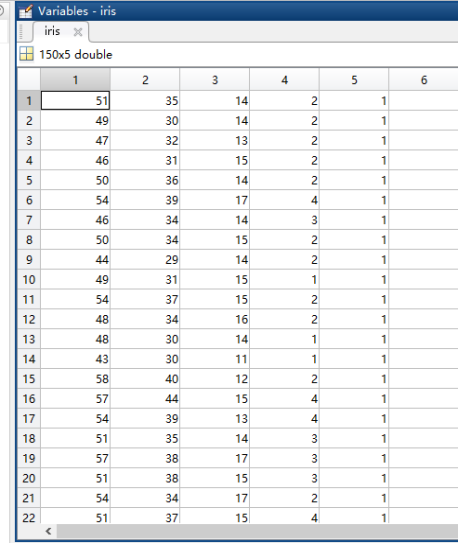2.数据预处理
（1）将原始iris数据集随机抽取118行作为训练数据集。再在这118行之外抽取32行（即剩余的32行）作为测试数据集。然后分别保存在trainData1.txt和testData1.txt中。
A1=randsample(150,118,false) ;
trainData1=iris(A1,:);
A2=randsample(150,32,false) ;
testData1=iris(A2,:);
save(‘trainData1.txt’,‘trainData1’,’-ascii’),
save(‘testData1.txt’,‘testData1’,’-ascii’),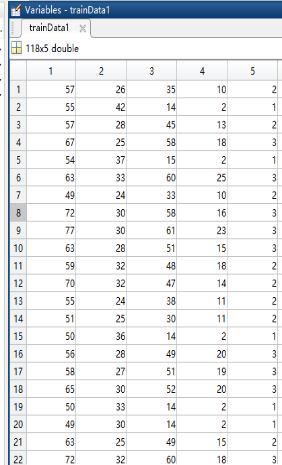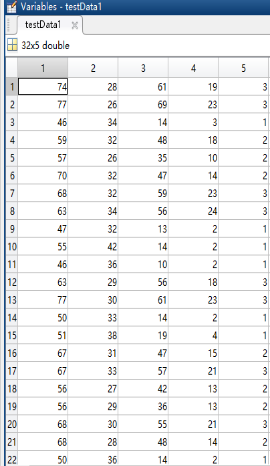（2）将训练数据每行相同格式的数据按语法读取150次，然后得到每列。
f1 f2 f3 f4是四个特征值,class是类别。
[f1,f2,f3,f4,class] = textread(‘trainData1.txt’ , ‘%f%f%f%f%f’,150);
（3）特征值归一化
把输入的4个属性矩阵归一化到[-1,1]，并保存到input矩阵中。
即下式为归一化公式：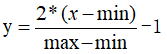[input,minI,maxI] = premnmx( [f1 , f2 , f3 , f4 ]’)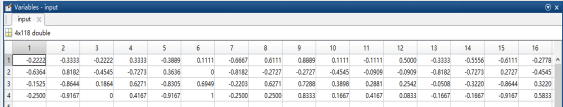（4）构造输出矩阵
s = length( class) ;  %得到类别矩阵长度：118
Output =zeros(s,3); %生成s行3列的全零阵:预先分配内存空间大小效率更高
for i = 1 : s
output( i , class( i )  ) = 1 ;
end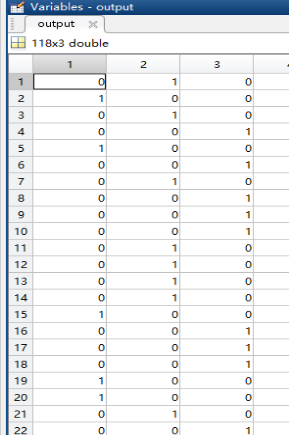output矩阵即为输出矩阵
例第一个样本即第一行：0 1 0 则表示属于第二类，而第二个样本即第二行： 1 0 0 则表示它属于第一类。
而 0 0 1则表示属于第三类。
3.创建神经网络
net = newff(minmax(input),[10 3] , { ‘logsig’ ‘logsig’ } , ‘traingdx’ );  minmax(input)：设定输入特征的范围—获取4个输入信号（存储在f1 f2 f3 f4中）的最大值和最小值；
{ ‘logsig’ ‘logsig’ }：隐层和输出层的传递函数–这里隐层和输出层传递函数都为logsig（S形传输函数）
‘traingdx’：表示学习规则采用的学习方法，即学习训练函数为traingdx（梯度下降）
4.设置训练参数
net.trainparam.show = 50 ;% 显示中间结果的周期
net.trainparam.epochs = 500 ;%最大迭代次数（学习次数）
net.trainparam.goal = 0.01;%神经网络训练的目标误差
net.trainParam.lr = 0.01 ;%学习速率
5.开始训练
将原先创建的神经网络以input为输入，output为目标函数的基础上训练epochs次即500次。
net = train( net, input , output’ ) ;
这里训练就结束了，以下开始测试。
6.开始测试
（1）读取测试数据
[t1, t2, t3, t4, c] = textread(‘testData1.txt’ , ‘%f%f%f%f%f’,150);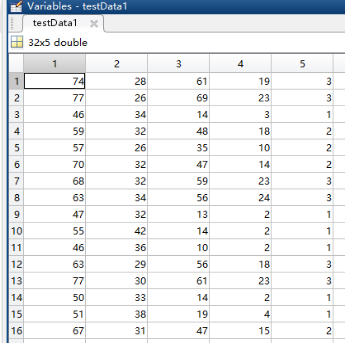（2）測试数据归一化
[testInput,minI,maxI] = premnmx( [t1 , t2 , t3 , t4 ]’)  ;（3）仿真
Y = sim( net , testInput ) ; %其中net为训练后得到的网络
Y是最终得到的输出结果，在该样本中，哪一类的值更大，则将该样本分给哪一类。
比如说第一列：就是第一个样本：（2.9182e-05,0.322，0.9606）中0.9606更大，那么将第一个样本分到第三类；再举例说第32列，即第32个样本：（0.0028,0.9408，0.0628）中0.9408更大，那么将第32个样本分给第二类。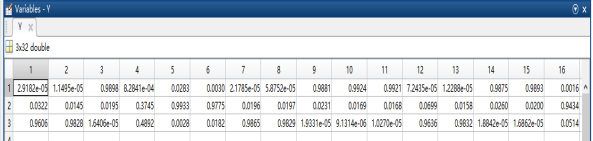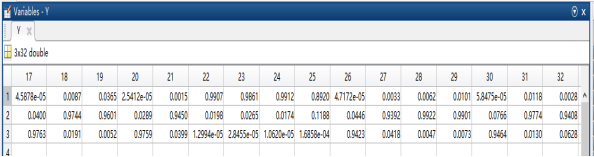7.统计识别正确率
[s1,s2] = size(Y) ; %返回矩阵的行-s1,列-s2
hitNum = 0 ;
for i = 1 : s2
[m,Index]= max(Y(:,i)); % m返回的是Y矩阵第i列中最大的数（归为类）
if(Index ==c(i))       % index返回的是Y矩阵第i列中最大的数下标
hitNum =hitNum + 1 ;
end
end
sprintf(‘识别率是 %3.3f%%’,100 * hitNum / s2 )
最终命令窗口得到识别的正确率如下：基本稳定在96.875%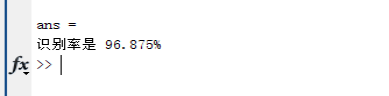三、实验结果分析及其对比（三层网络结构）
1.改变输出层的传递函数 {‘logsig’ ‘logsig’}
（1）左图输出层为s型传递函数即logsig  ，右图为线性传递函数即purelin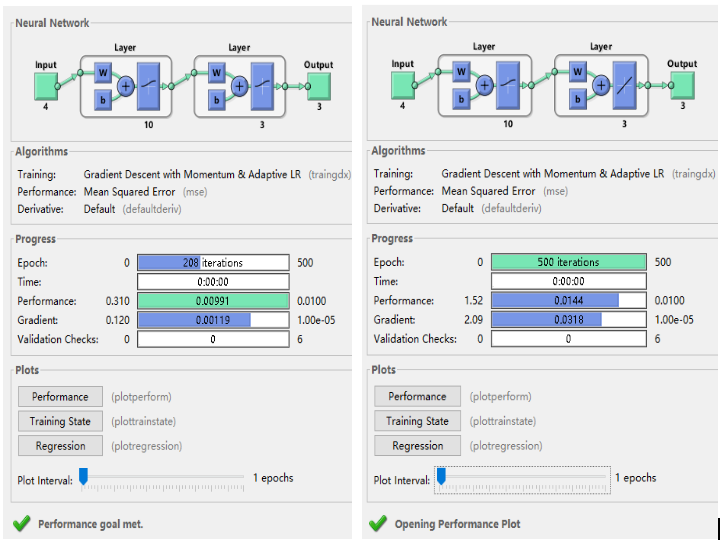Performance：性能-用mse评估，进度条右边是设定的均方误差，即通过参数net.trainparam.goal设定。
Gradient：梯度-当梯度值达到了1.00e-05时，则停止训练
Validation check：泛化能力检查-若连续6次训练误差不降反升（误差不再减小，不会再有更好的效果了），则停止训练。
（2）训练集性能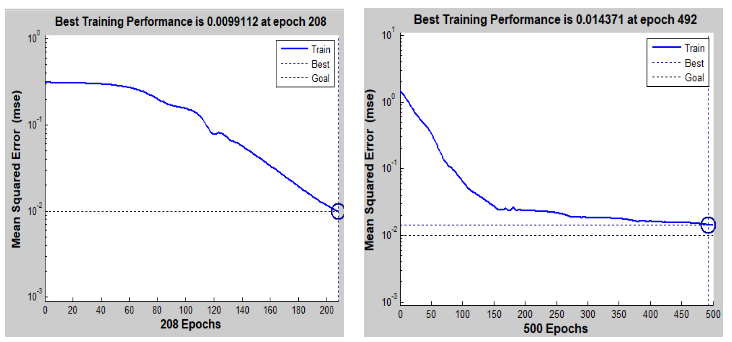从纵坐标就可以知道，它通过均方差开衡量网络的性能。由图可看到随着迭代次数的增大，均方误差越来越小，性能越好。
总结：改变输出层传递函数对训练周期、训练性能有一定影响，本例中左图迭代到208次收敛，均方误差0.00991；而右图迭代到492次才收敛，均方误差0.0144，超过了目标误差0.01；所以输出层为s型传递函数要优于线性传递函数。
2.改变隐层的传递函数 {‘losig’ ‘losig’}
（1）左图隐层为s型传递函数即logsig  ，右图隐层为双曲正切S型传递函数即tansig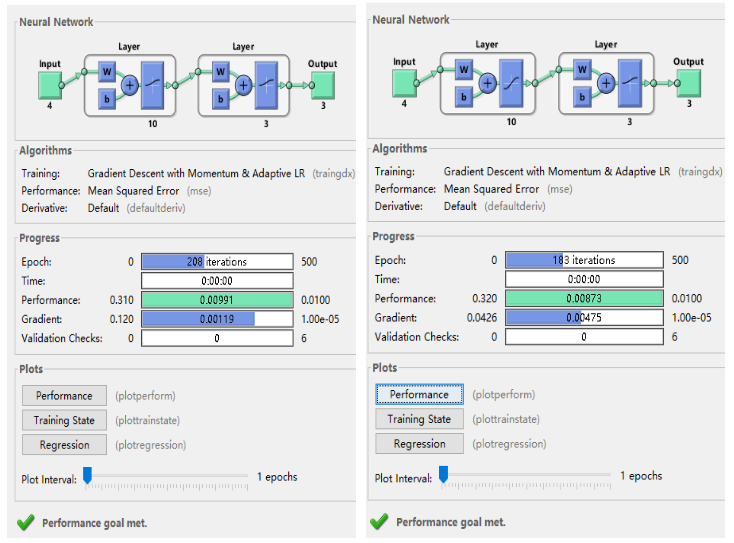（2）训练集性能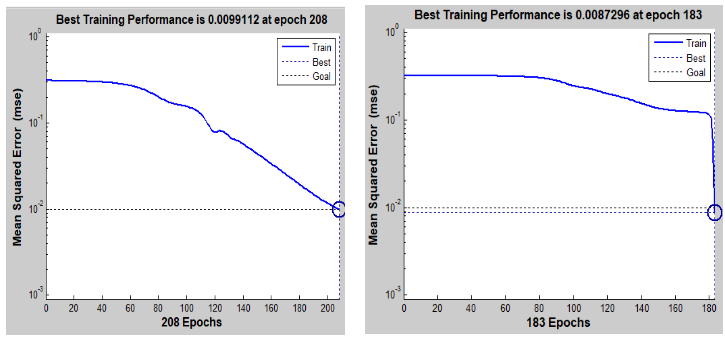总结：改变隐层传递函数对训练的性能、训练周期都有影响，本例中左图迭代到208次收敛，均方误差0.00991；而右图迭代到183次收敛，均方误差0.00873；所以隐层传递函数使用双曲正切s型传递函数要比S型传递函数更好一些。
3.改变学习率
（1）左图学习率为0.01 ，右图为0.05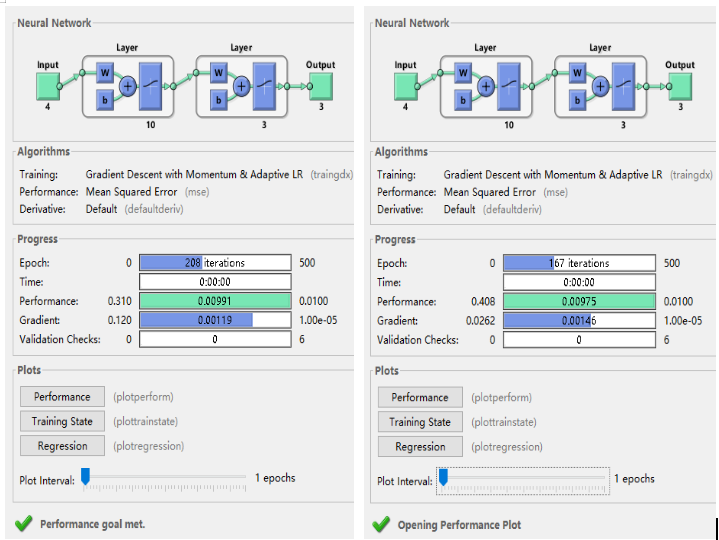（2）训练集性能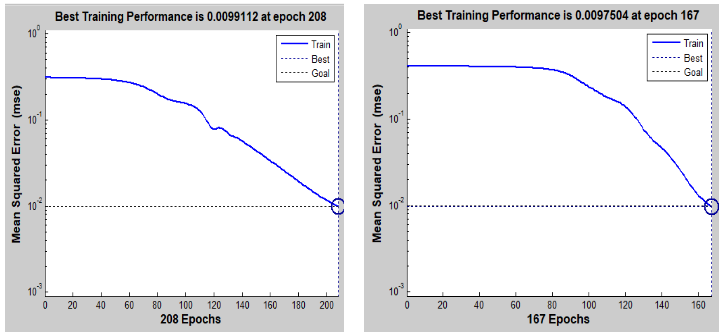总结：学习速率的选取大了可能导致系统不稳定，小了会导致训练周期过长、收敛慢，达不到要求的误差。一般通过观察误差下降曲线来判断。同时，由于网络规模大小的不同，学习率选择应当针对其进行调整。
四、代码（matlab实现）
参考别人代码，本人仅仅做了一点改动，并加以分析。
%读取训练数据
clear
clc
load iris.dat;

%随机提取完数据并保存之后，要注释掉以下这6行代码
%因为每次训练都必须是同样的训练集和测试集，不能每训练一次随机提取一次。
%A1=randsample(150,118,false) ;
%trainData1=iris(A1,:);
%A2=randsample(150,32,false) ;
%testData1=iris(A2,:);
%save('trainData1.txt','trainData1','-ascii'),
%save('testData1.txt','testData1','-ascii'),

%f1 f2 f3 f4是四个特征值
[f1,f2,f3,f4,class] = textread('trainData1.txt' , '%f%f%f%f%f',150);
%特征值归一化
[input,minI,maxI] = premnmx( [f1 , f2 , f3 , f4 ]')  ;
%构造输出矩阵
s = length( class) ;
output = zeros( s , 3  ) ;
for i = 1 : s
output( i , class( i )  ) = 1 ;
end
%创建神经网络
net = newff( minmax(input) , [10 3] , { 'logsig' 'logsig' } , 'traingdx' ) ;
%{
minmax(input)：获取4个输入信号（存储在f1 f2 f3 f4中）的最大值和最小值；
[10,3]：表示使用2层网络，第一层网络节点数为10，第二层网络节点数为3；
{ 'logsig' 'purelin' }：
表示每一层相应神经元的激活函数；
即：第一层神经元的激活函数为logsig（对数S形转移函数），第二层为purelin（线性函数）
'traingdx'：表示学习规则采用的学习方法为traingdx（梯度下降自适应学习率训练函数）
%}
%设置训练參数
net.trainparam.show = 50 ;% 显示中间结果的周期
net.trainparam.epochs = 500 ;%最大迭代次数（学习次数）
net.trainparam.goal = 0.01;%神经网络训练的目标误差
net.trainParam.lr = 0.01 ;%学习速率（Learning rate）
%开始训练
%其中input为训练集的输入信号，对应output为训练集的输出结果
net = train( net, input , output' ) ;

%================================训练完成====================================%
%% 读取测试数据并归一化
%=============================接下来进行测试=================================%
%读取测试数据
[t1, t2, t3, t4, c] = textread('testData1.txt' , '%f%f%f%f%f',150);
%測试数据归一化
[testInput,minI,maxI] = premnmx( [t1 , t2 , t3 , t4 ]')  ;
%仿真
%其中net为训练后得到的网络，返回的Y为
Y = sim( net , testInput ) ;
%统计识别正确率
[s1 , s2] = size( Y ) ;
hitNum = 0 ;
for i = 1 : s2
[m , Index] = max( Y( : ,  i ) ) ;
if( Index  == c(i)   )
hitNum = hitNum + 1 ;
end
end
sprintf('识别率是 %3.3f%%',100 * hitNum / s2 )

数据集链接我已上传，好久没看评论！！！鸢尾花数据集


展开全文人工智能
• 本代码（MATLAB）可直接运行，对Iris鸢尾花数据分类，准确率96%左右，随机2/3数据训练，1/3数据测试。对于新手有帮助，多为矩阵运算。
• 摘要：本文主要介绍如何用麻雀算法优化BP神经网络，利用鸢尾花数据，做一个简单的讲解。 1.鸢尾花iris数据介绍 本案例利用matlab公用的iris鸢尾花数据，作为测试数据，iris数据是特征为4维，类别为3个类别。数据格式...算法 深度学习 人工智能 机器学习
• matlab实现用Bp神经网络对iris数据集进行分类（以及影响分类性能的参数条件）这篇文章的数据集
• 下面给出matlab解决鸢尾花分类问题的代码。 神经网络参数预测 function [ w1,w2] = NN( x, y, lamda,units, alpha ) % 实现一个包含一个隐含层，隐含层中非偏置单元数为units的bp神经网络，用于分类问题 % 激活函数...机器学习
• matlab和python实现创建BP神经网络，对鸢尾花数据集进行分类
• 数据挖掘结课设计，老师要求剖析一个分类程序，一开始找了个用Matlab实现的，也就是我上一篇博客所写的。有点心虚，毕竟一共才40多行代码，问...又在网上找了篇文章：BP算法实例—鸢尾花分类（pyhton） https://bl...python
• 学了一段时间python，自己尝试编写的第一个算法——BP神经网络，并将应用到鸢尾花数据集上，数据集连接如下： 鸢尾花数据集iris0.txt 数据集集已经进行了处理，属于纯纯的0-1问题分类（二分类问题）， 先说一说在...python 机器学习
• 26 有导师学习神经网络分类——鸢尾花种类识别（郁磊） 27 无导师学习神经网络分类——矿井突水水源判别（郁磊） 28 支持向量机的分类——基于乳腺组织电阻抗特性的乳腺癌诊断（郁磊） 29 支持向量机的回归...
• 26 有导师学习神经网络分类——鸢尾花种类识别（郁磊） 有导师学习神经网络以其良好的学习能力广泛应用于各个领域中，其不仅可以解决拟 合回归问题，亦可以用于模式识别、分类识别。本章将继续介绍两种典型的有...
• 本文件含一些数据集及matlab代码。分别ORL人脸图像（含有数据集）、ANN神经网络入门-分类问题（含鸢尾花数据集）、Iris（鸢尾花数据集）等。# matlab神经网络分类鸢尾花matlab 订阅